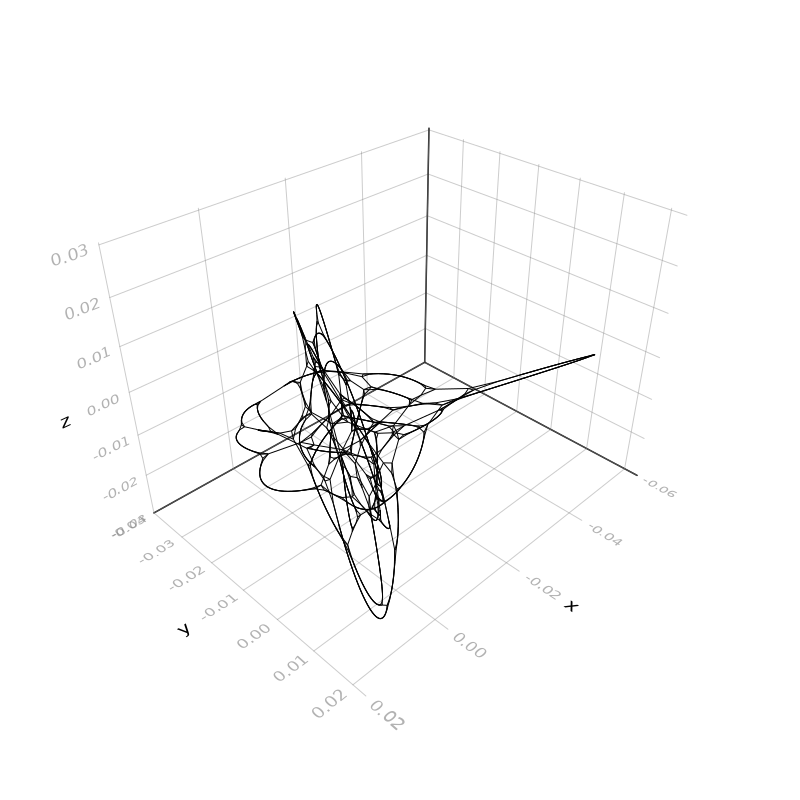# NetworkLayout

This is the Documentation for NetworkLayout.

All example images on this page are created using Makie.jl and the graphplot recipe from GraphMakie.jl.

using CairoMakie
using NetworkLayout
using GraphMakie, Graphs

# Basic Usage & Algorithms

All of the algorithms follow the Layout Interface. Each layout algorithm is represented by a type LayoutAlgorithm <: AbstractLayout. The parameters of each layout can be set with keyword arguments. The LayoutAlgorithm object itself is callable and transforms the adjacency matrix and returns a list of Point{N,T} from GeometryBasics.jl.

alg = LayoutAlgorithm(; p1="foo", p2=:bar)
positions = alg(adj_matrix)

Each of the layouts comes with a lowercase function version:

positions = layoutalgorithm(adj_matrix; p1="foo", b2=:bar)

Instead of using the adjacency matrix you can use AbstractGraph types from Graphs.jl directly.

g = complete_graph(10)
positions = layoutalgorithm(g)

## Scalable Force Directed Placement

NetworkLayout.SFDPType
SFDP(; kwargs...)(adj_matrix)
sfdp(adj_matrix; kwargs...)

Using the Spring-Electric model suggested by Yifan Hu. Forces are calculated as:

    f_attr(i,j) = ‖xi - xj‖ ² / K ,    i<->j
f_repln(i,j) = -CK² / ‖xi - xj‖ ,  i!=j

Takes adjacency matrix representation of a network and returns coordinates of the nodes.

Keyword Arguments

• dim=2, Ptype=Float64: Determines dimension and output type Point{dim,Ptype}.

• tol=1.0: Stop if position changes of last step Δp <= tol*K for all nodes

• C=0.2, K=1.0: Parameters to tweak forces.

• iterations=100: maximum number of iterations

• initialpos=Point{dim,Ptype}[]

Provide list of initial positions. If length does not match Network size the initial positions will be truncated or filled up with random values between [-1,1] in every coordinate.

• seed=1: Seed for random initial positions.

source

### Example

g = wheel_graph(10)
layout = SFDP(Ptype=Float32, tol=0.01, C=0.2, K=1)
f, ax, p = graphplot(g, layout=layout)
hidedecorations!(ax); hidespines!(ax); ax.aspect = DataAspect(); f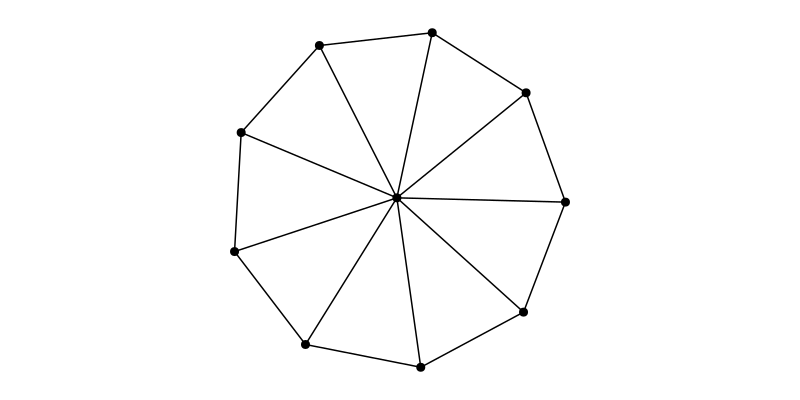### Iterator Example

iterator = LayoutIterator(layout, g)
record(f, "sfdp_animation.mp4", iterator; framerate = 10) do pos
p[:node_pos][] = pos
autolimits!(ax)
end

## Buchheim Tree Drawing

NetworkLayout.BuchheimType
Buchheim(; kwargs...)(adj_matrix)
buchheim(adj_list; kwargs...)

Using the algorithm proposed in the paper, "Improving Walker's Algorithm to Run in Linear Time" by Christoph Buchheim, Michael Junger, Sebastian Leipert

Takes adjacency matrix or list representation of given tree and returns coordinates of the nodes.

Keyword Arguments

• Ptype=Float64: Determines the output type Point{2,Ptype}.

• nodesize=Float64[]

Determines the size of each of the node. If network size does not match the length of nodesize fill up with ones or truncate given parameter.

source

### Example

adj_matrix = [0 1 1 0 0 0 0 0 0 0;
0 0 0 0 1 1 0 0 0 0;
0 0 0 1 0 0 1 0 1 0;
0 0 0 0 0 0 0 0 0 0;
0 0 0 0 0 0 0 1 0 1;
0 0 0 0 0 0 0 0 0 0;
0 0 0 0 0 0 0 0 0 0;
0 0 0 0 0 0 0 0 0 0;
0 0 0 0 0 0 0 0 0 0;
0 0 0 0 0 0 0 0 0 0]
layout = Buchheim()
f, ax, p = graphplot(g, layout=layout)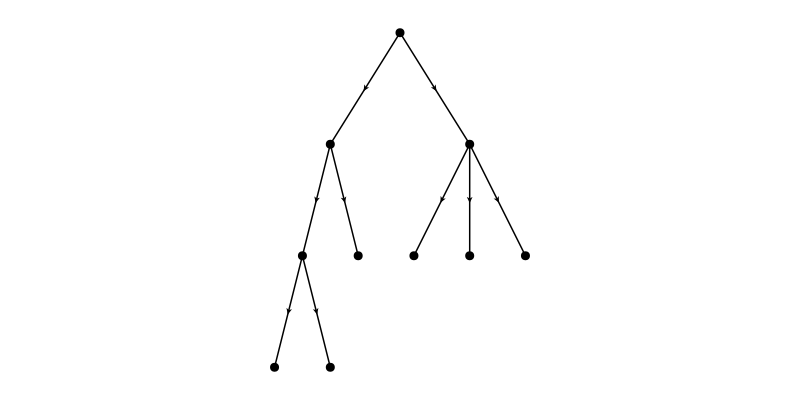## Spring/Repulsion Model

NetworkLayout.SpringType
Spring(; kwargs...)(adj_matrix)
spring(adj_matrix; kwargs...)

Use the spring/repulsion model of Fruchterman and Reingold (1991) with

• Attractive force: f_a(d) = d^2 / k
• Repulsive force: f_r(d) = -k^2 / d

where d is distance between two vertices and the optimal distance between vertices k is defined as C * sqrt( area / num_vertices ) where C is a parameter we can adjust

Takes adjacency matrix representation of a network and returns coordinates of the nodes.

Keyword Arguments

• dim=2, Ptype=Float64: Determines dimension and output type Point{dim,Ptype}.

• C=2.0: Constant to fiddle with density of resulting layout

• iterations=100: maximum number of iterations

• initialtemp=2.0: Initial "temperature", controls movement per iteration

• initialpos=Point{dim,Ptype}[]

Provide list of initial positions. If length does not match Network size the initial positions will be truncated or filled up with random values between [-1,1] in every coordinate.

• seed=1: Seed for random initial positions.

source

### Example

g = smallgraph(:cubical)
layout = Spring(Ptype=Float32)
f, ax, p = graphplot(g, layout=layout)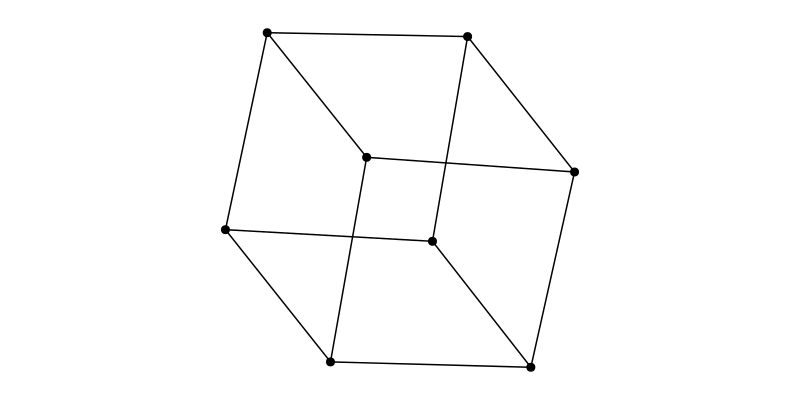### Iterator Example

iterator = LayoutIterator(layout, g)
record(f, "spring_animation.mp4", iterator; framerate = 10) do pos
p[:node_pos][] = pos
autolimits!(ax)
end

## Stress Majorization

NetworkLayout.StressType
Stress(; kwargs...)(adj_matrix)
stress(adj_matrix; kwargs...)

Compute graph layout using stress majorization. Takes adjacency matrix representation of a network and returns coordinates of the nodes.

Inputs:

• adj_matrix: Matrix of pairwise distances.

Keyword Arguments

• dim=2, Ptype=Float64: Determines dimension and output type Point{dim,Ptype}.

• iterations=:auto: maximum number of iterations (:auto means 400*N^2 where N are the number of vertices)

• abstols=(√(eps(Float64)))

Absolute tolerance for convergence of stress. The iterations terminate if the difference between two successive stresses is less than abstol.

• reltols=(√(eps(Float64)))

Relative tolerance for convergence of stress. The iterations terminate if the difference between two successive stresses relative to the current stress is less than reltol.

• abstolx=(√(eps(Float64)))

Absolute tolerance for convergence of layout. The iterations terminate if the Frobenius norm of two successive layouts is less than abstolx.

• weights=Array{Float64}(undef, 0, 0)

Matrix of weights. If empty (i.e. not specified), defaults to weights[i,j] = δ[i,j]^-2 if δ[i,j] is nonzero, or 0 otherwise.

• initialpos=Point{dim,Ptype}[]

Provide list of initial positions. If length does not match Network size the initial positions will be truncated or filled up with random normal distributed values in every coordinate.

• seed=1: Seed for random initial positions.

Reference:

The main equation to solve is (8) of:

@incollection{
author = {Emden R Gansner and Yehuda Koren and Stephen North},
title = {Graph Drawing by Stress Majorization}
year={2005},
isbn={978-3-540-24528-5},
booktitle={Graph Drawing},
seriesvolume={3383},
series={Lecture Notes in Computer Science},
editor={Pach, J'anos},
doi={10.1007/978-3-540-31843-9_25},
publisher={Springer Berlin Heidelberg},
pages={239--250},
}
source

### Example

g = SimpleGraph(936)
for l in eachline(joinpath(@__DIR__,"..","..","test","jagmesh1.mtx"))
s = split(l, " ")
src, dst = parse(Int, s), parse(Int, s)
src != dst && add_edge!(g, src, dst)
end

layout = Stress(Ptype=Float32)
f, ax, p = graphplot(g; layout=layout, node_size=3, edge_width=1)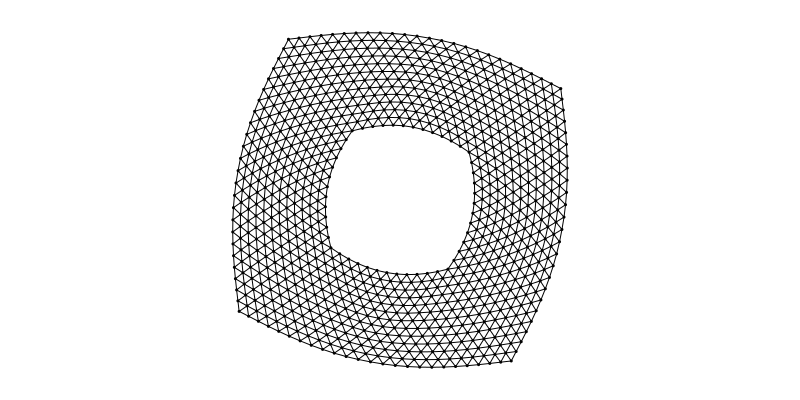### Iterator Example

iterator = LayoutIterator(layout, g)
record(f, "stress_animation.mp4", iterator; framerate = 7) do pos
p[:node_pos][] = pos
autolimits!(ax)
end

## Shell/Circular Layout

NetworkLayout.ShellType
Shell(; kwargs...)(adj_matrix)
shell(adj_matrix; kwargs...)

Position nodes in concentric circles. Without further arguments all nodes will be placed on a circle with radius 1.0. Specify placement of nodes using the nlist argument.

Takes adjacency matrix representation of a network and returns coordinates of the nodes.

Keyword Arguments

• Ptype=Float64: Determines the output type Point{2,Ptype}.

• nlist=Vector{Int}[]

Vector of Vector of node indices. Tells the algorithm, which nodes to place on which shell from inner to outer. Nodes which are not present in this list will be place on additional outermost shell.

This function started as a copy from IainNZ's GraphLayout.jl

source
g = smallgraph(:petersen)
layout = Shell(nlist=[6:10,])
f, ax, p = graphplot(g, layout=layout)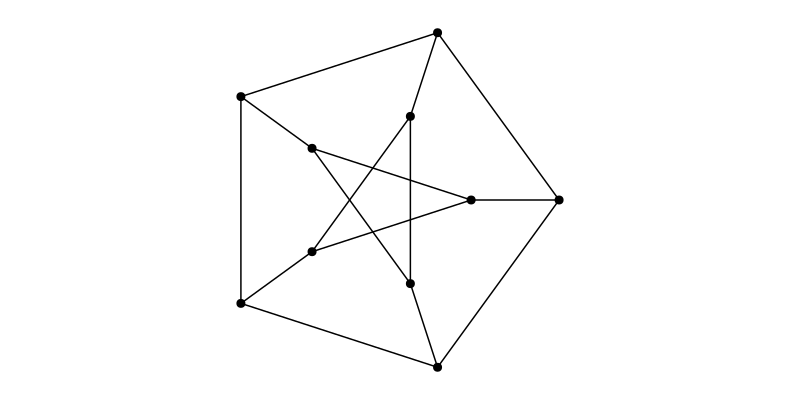## SquareGrid Layout

NetworkLayout.SquareGridType
SquareGrid(; kwargs...)(adj_matrix)
squaregrid(adj_matrix; kwargs...)

Position nodes on a 2 dimensional rectagular grid. The nodes are palced in order from upper left to lower right. To skip positions see skip argument.

Takes adjacency matrix representation of a network and returns coordinates of the nodes.

Keyword Arguments

• Ptype=Float64: Determines the output type Point{2,Ptype}
• cols=:auto: Columns of the grid, the rows are determined automatic. If :auto the layout will be square-ish.
• dx=Ptype(1), dy=Ptype(-1): Ofsets between rows/cols.
• skip=Tuple{Int,Int}[]: Specify positions to skip when placing nodes. skip=[(i,j)] means to keep the position in the i-th row and j-th column empty.
source
g = Grid((12,4))
layout = SquareGrid(cols=12)
f, ax, p = graphplot(g, layout=layout, nlabels=repr.(1:nv(g)), nlabels_textsize=10, nlabels_distance=5)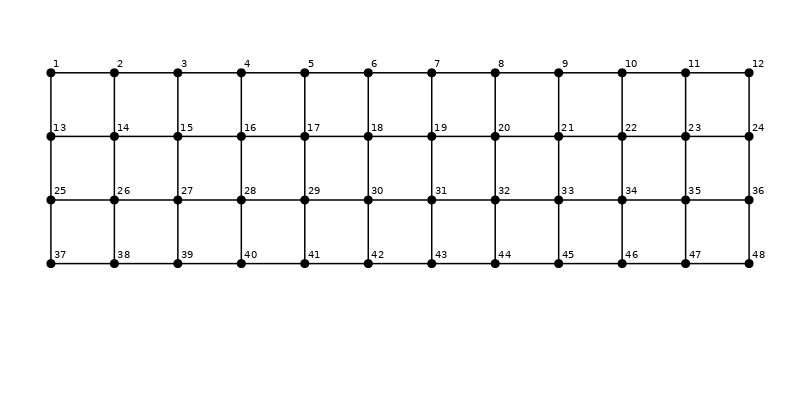## Spectral Layout

NetworkLayout.SpectralType
Spectral(; kwargs...)(adj_matrix)
spectral(adj_matrix; kwargs...)

This algorithm uses the technique of Spectral Graph Drawing, which is an under-appreciated method of graph layouts; easier, simpler, and faster than the more common spring-based methods. For reference see Yehuda Koren, 2002.

Takes adjacency matrix representation of a network and returns coordinates of the nodes.

Keyword Arguments

• dim=3, Ptype=Float64: Determines dimension and output type Point{dim,Ptype}.
• nodeweights=Float64[]: Vector of weights. If network size does not match the length of nodesize use ones instead.
source
g = watts_strogatz(1000, 5, 0.03; seed=5)
layout = Spectral(dim=2)
f, ax, p = graphplot(g, layout=layout, node_size=0.0, edge_width=1.0)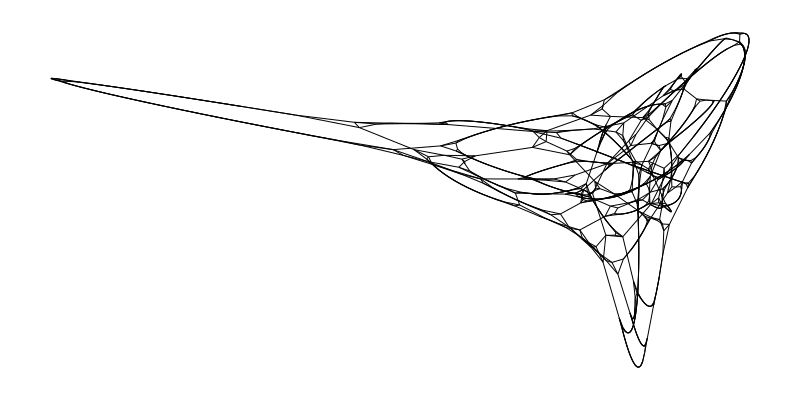layout = Spectral()
f, ax, p = graphplot(g, layout=layout, node_size=0.0, edge_width=1.0)